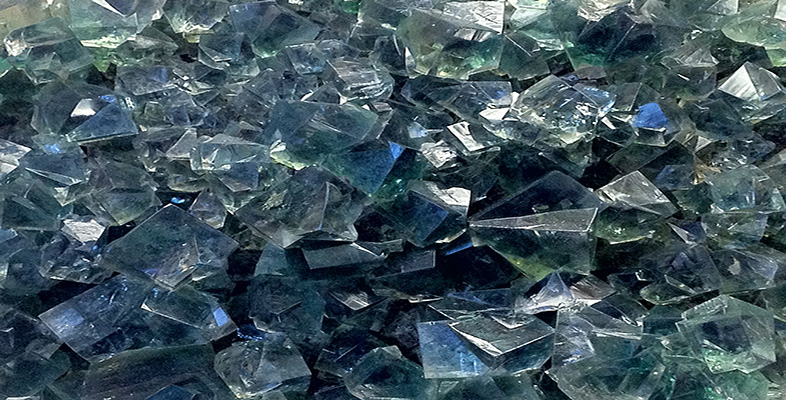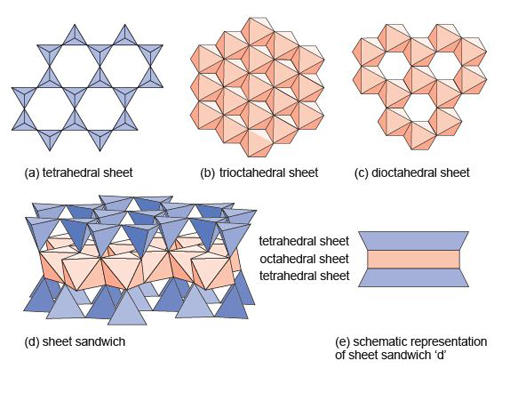Science, Maths & Technology

### Become an OU studentAn introduction to minerals and rocks under the microscope

Start this free course now. Just create an account and sign in. Enrol and complete the course for a free statement of participation or digital badge if available.

# 3.5 Sheet silicate minerals

The sheet silicates comprise a large number of different minerals, including micas. They all share the same basic building blocks: a tetrahedral sheet (Figure 48a), and one of two kinds of octahedral sheet.Figure 48 Building blocks of sheet silicate minerals. (a) Part of a sheet made up of SiO4 tetrahedra. (b) Part of a Mg(OH)2trioctahedral sheet, where every oxygen atom is shared between three octahedra. (c) Part of an Al(OH)3dioctahedral sheet, where every oxygen atom is shared between two octahedra. (d) An oblique view of a sheet sandwich, formed by two tetrahedral sheets bonded to an octahedral sheet. (e) A schematic representation of the sheet sandwich in (d).

The octahedral sheets can be thought of as layers of metal hydroxides. One kind of octahedral sheet is effectively a layer of magnesium hydroxide, Mg(OH)2, where each magnesium atom is bonded to six OH groups at the corners of an octahedron. The octahedra are arranged in a plane, sharing edges, to form a trioctahedral sheet as each oxygen atom is shared between three octahedra (Figure 48b).

The other kind of octahedral sheet contains aluminium instead of magnesium, and is effectively a layer of aluminium hydroxide, Al(OH)3. In this case each oxygen atom is shared between two octahedra, hence this is a dioctahedral sheet (Figure 48c), and it leaves distinct holes in the structure. In sheet silicates, the sheets are combined to form composite structures: for example an octahedral sheet sandwiched between two tetrahedral sheets as shown in Figure 48d, and more schematically in Figure 48e.

A property common to all sheet silicate minerals is their tendency to be soft, with near-perfect cleavage parallel to the sheets. They form distinctive, platy or flake-like crystals. With (OH) groups as part of their structure, they are all hydrous minerals.